Do you like the article?
Share it with others -

Use new possibilities of MetaTrader 5

# Creating an Information Board Using the Standard Library Classes and Google Chart API

5 July 2010, 12:513
6 449

### Introduction

To make life easier for programmers of the MQL5 language, designers have created a Standard Library, which covers almost all of the API MQL5 functions, and makes working with them much easier and convenient. This article will attempt to create an information board, with a maximum number of classes used by the standard library.

### 1. Overview of the Standard Library classes

So, what exactly is this library? The Documentation section of the website states that it is composed of:

The files, containing the codes of all classes, are located in the MQL5/Include folder. When viewing the library code, you will notice, that it provides only the classes, but not the functions. Consequently, to use it, you must have some knowledge of object-oriented programming (OOP).

All of the library classes (except trading ones) stem from CObject base class. To show it, we'll try to construct a Class diagram, since we have everything that this requires - the base class and its heirs. Since the MQL5 language is basically a subset of C++, let us use the IBM Rational Rose instrument, which provides tools for reverse -engineering of C++ projects, for the automatic construction of the diagram.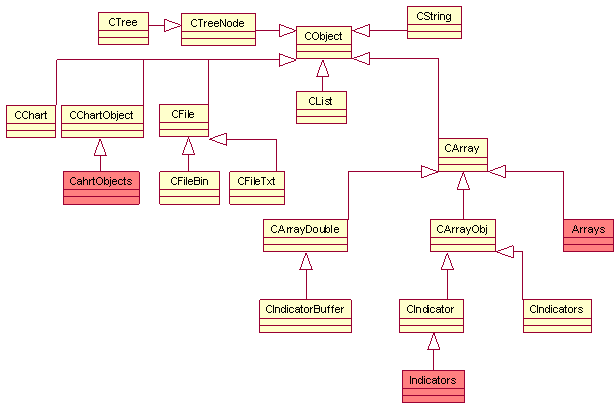Figure 1. Diagram of the Standard Library classes

We will not be showing the class properties and methods, due to the cumbersome diagrams that we would obtain. We will also omit aggregations, since they are of no importance to us. As a result, we are left only with generalizations (inheritances), which allow us to find out what properties and methods the classes obtain.

As can be seen from the diagram, each library component that works with lines, files, charts, graphic objects, and arrays, has its own base class (CString, CFile, CChart, CChartObject and CArray, Respectively), inherited from the CObject. The base class for working with indicators CIndicator and his auxiliary CIndicators class are inherited from the CArrayObj, while the access to indicator buffer class CIndicatorBuffer is inherited from the CArrayDouble.

Crimson color in the diagram marks the non-existent in actuality classes, indicators, arrays, and ChartObjects - they are sets, which include classes for working with indicators, arrays, and graphic objects. Since there is a large number of them, and they are inherited from a single parent, I allowed for some simplification, so as not to clutter the diagram. For example, the Indicator includes CiDEMA, CiStdDev, etc.

It is also worth noting that the class diagram can also be constructed using the automatic creation of Doxygen documentation system. It is somewhat easier to do this in this system rather than in Rational Rose. More about Doxygen can be found in the article Auto-Generated Documentation for MQL5 Code.

### 2. The problem

Let's try to create an information table with the maximum number of  the Standard Library classes.

What will the board display? Something like a detailed report of MetaTrader 5, ie: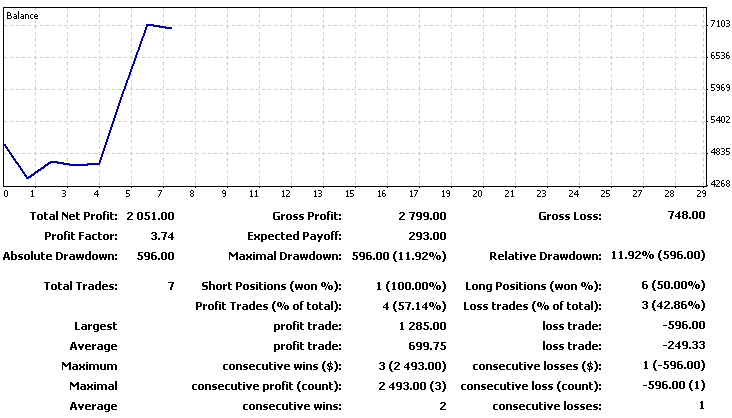Figure 2. The appearance of the detailed report

As we can see, the report displays a balance chart and some trading figures. More information about the methods for calculating these indicators can be found in the article What the Numbers in the Expert Testing Report Mean.

Since the board is used purely for informational purposes, and does not perform any trading operations, it will be best to implement it as an indicator, in a separate window, in order to avoid closing the actual chart. Moreover, placing it in a sub-window allows for easy scaling, and even closing the board with a single motion of your mouse.

You may also want to complement the report with a pie chart, which will display the number of transactions made on the instrument, relative to the total number of transactions.

### 3. Designing the interface

We have defined our objectives - we need a detailed report in the sub-window of the main chart.

We implement our information board as a class. Let's begin:

```//+------------------------------------------------------------------+
///The Board class
//+------------------------------------------------------------------+
class Board
{
//protected data
protected:
///number of the sub-window where the board will be stored
int               wnd;
///array with the deals data
CArrayObj        *Data;
///array with the balance data
CArrayDouble      ChartData;
///array with elements of the interface
CChartObjectEdit  cells;
///object for working with the chart
CChart            Chart;
///object for working with the balance chart
CChartObjectBmpLabel BalanceChart;
///object for working with the pie chart
CChartObjectBmpLabel PieChart;
///data for the pie chart
PieData          *pie_data;
//private data and methods
private:
double            net_profit;      //these variables will store the calculated characteristics
double            gross_profit;
double            gross_loss;
double            profit_factor;
double            expected_payoff;
double            absolute_drawdown;
double            maximal_drawdown;
double            maximal_drawdown_pp;
double            relative_drawdown;
double            relative_drawdown_pp;
int               total;
int               short_positions;
double            short_positions_won;
int               long_positions;
double            long_positions_won;
int               maximum_consecutive_wins;
double            maximum_consecutive_wins_usd;
int               maximum_consecutive_losses;
double            maximum_consecutive_losses_usd;
int               maximum_consecutive_profit;
double            maximum_consecutive_profit_usd;
int               maximum_consecutive_loss;
double            maximum_consecutive_loss_usd;
int               average_consecutive_wins;
int               average_consecutive_losses;

///method of obtaining data about the deals and the balance
void              GetData();

///method of calculating the characteristics
void              Calculate();

///method of chart construction
void              GetChart(int X_size,int Y_size,string request,string file_name);

///method of request to Google Charts API

///method of obtaining the optimum font size
int               GetFontSize(int x,int y);
string            colors;  //array with text presentation of colors
//public methods
public:
///constructor
void              Board();
///destructor
void             ~Board();
///method for board update
void              Refresh();
///method for creating interface elements
void              CreateInterface();
};
```

The protected class data are the interface elements and deal, balance, and pie chart data (the class PieData will be discussed below). Trading indicators and some methods are private. They are private  because the user should not have direct access to them, they are calculated within the class, and they can be counted only through calling the appropriate public method.

Also the methods of the interface creation and the calculation of indicators are private, since here you need to endure a rigorous sequence of method calls. For example, it is impossible to calculate the indicators without having the data for calculation, or to update the interface, without having to create it beforehand. Thus, we will not allow the user to "shoot himself in the foot".

Let us immediately deal with constructors and destructors of a class, so we don't have to return to them later:

```//+------------------------------------------------------------------+
///Constructor
//+------------------------------------------------------------------+
void Board::Board()
{
Chart.Attach();                               //attach the current chart to the class instance
wnd=ChartWindowFind(Chart.ChartId(),"IT");    //find the indicator window
Data = new CArrayObj;                         //creating the CArrayObj class instance
pie_data=new PieData;                         //creating the PieData class instance
//fill colors array
colors="003366"; colors="00FF66"; colors="990066";
colors="FFFF33"; colors="FF0099"; colors="CC00FF";
colors="990000"; colors="3300CC"; colors="000033";
colors="FFCCFF"; colors="CC6633"; colors="FF0000";
}
//+------------------------------------------------------------------+
///Destructor
//+------------------------------------------------------------------+
void Board::~Board()
{
if(CheckPointer(Data)!=POINTER_INVALID) delete Data;   //delete the deals data
if(CheckPointer(pie_data)!=POINTER_INVALID) delete pie_data;
ChartData.Shutdown();    //and balance data
Chart.Detach();          //detach from the chart
for(int i=0;i<10;i++)    //delete all interface elements
for(int j=0;j<6;j++)
cells[i][j].Delete();
BalanceChart.Delete();   //delete the balance chart
PieChart.Delete();       //and pie chart
}
```

In the constructor, we will tie an object of type CChart to the current chart with the help of its Attach() method. The Detach() method, called in the destructor, will untie the chart from the object. Data object, which is a pointer to an object of CArrayObj type, received the object's address, created dynamically using the new operation and removed in destructor using delete operato. Do not forget to check for the object presence usingg the CheckPointer() before deleting, otherwise an error will occur.

More information about the CArrayObj class will be provided further. The Shutdown() method of CArrayDouble class just like any other class is inherited from CArray class (see diagram of classes) will clear and free up the memory, occupied by the object. The Delete() method of CChartObject class heirs removes the object from the chart.

Thus, the constructor allocates the memory and the destructor frees it, and removes the graphical objects, created by the class.

Let's now deal with the interface. As stated above, the CreateInterface() method creates an interface of the board:

```//+------------------------------------------------------------------+
///CreateInterface function
//+------------------------------------------------------------------+
void Board::CreateInterface()
{
//retrieve the width
int x_size=Chart.WidthInPixels();
//and the height of the indicator window
int y_size=Chart.GetInteger(CHART_HEIGHT_IN_PIXELS,wnd);

//calculate, how much space will the balance chart take up
double chart_border=y_size*(1.0-(Chart_ratio/100.0));

if(Chart_ratio<100)//if the balance chart is taking up the entire table
{
for(int i=0;i<10;i++)//create columns
{
for(int j=0;j<6;j++)//and rows
{
cells[i][j].Create(Chart.ChartId(),"InfBoard "+IntegerToString(i)+" "+IntegerToString(j),
wnd,j*(x_size/6.0),i*(chart_border/10.0),x_size/6.0,chart_border/10.0);
//set selectable property to false
cells[i][j].Selectable(false);
//set font size
cells[i][j].FontSize(GetFontSize(x_size/6.0, chart_border/10.0));
cells[i][j].Font("Arial");    //font name
cells[i][j].Color(text_color);//font color
}
}
}

if(Chart_ratio>0)//if the balance chart is required
{
//create a balance chart
BalanceChart.Create(Chart.ChartId(), "InfBoard chart", wnd, 0, chart_border);
//set selectable property to false
BalanceChart.Selectable(false);
//create a pie chart
PieChart.Create(Chart.ChartId(), "InfBoard pie_chart", wnd, x_size*0.75, chart_border);
PieChart.Selectable(false);//set selectable property to false
}

Refresh();//refresh the board
}```

For a compact arrangement of all the elements, first, using the WidthInPixels() and GetInteger() methods of the class CChart, find out the length and width of the indicator subwindow, in which the board will be located. Then we create the cells, which will include the values of the indicators, using the Create() method of the CChartObjectEdit class (creates the "input field"), all heirs have this method of CChartObject.

Note how convenient it is to use the Standard Library for operations of this type. Without it we would have to create each object, using the function ObjectCreate, and set the properties of objects, using such functions as ObjectSet, which would lead to code redundancy. And when later we would want to change the properties of the objects, it would be necessary to carefully control the objects' names in order to avoid confusion. Now we can simply create an array of graphic objects, and look through it as we desire.

In addition, we can get/set properties of objects using one function, if it was overloaded creators of the class, such as Color() method of CChartObject class. When called with the parameters it sets thems, without parameters - it returns the object color. Place the pie chart next to the balance chart, it will take up a quarter of the total width of the screen.

Refresh method() updates the board. What does this update consist of? We need to count up the indicators, enter them into the graphic objects, and rescale the board, if the size of the window in which it is located had been altered. The board should take up the entire free space of the window.

```//+------------------------------------------------------------------+
///Function of the board updating
//+------------------------------------------------------------------+
void Board::Refresh()
{
//check the server connection status
//check the permission for importing functions from DLL
//calculate the characteristics
Calculate();
//retrieve the width
int x_size=Chart.WidthInPixels();
//and the height of the indicator window
int y_size=Chart.GetInteger(CHART_HEIGHT_IN_PIXELS,wnd);
//calculate how much space the balance chart will take up
double chart_border=y_size*(1.0-(Chart_ratio/100.0));

string captions= //array with signatures of interface elements
{
{"Total Net Profit:"," ","Gross Profit:"," ","Gross Loss:"," "},
{"Profit Factor:"," ","Expected Payoff:"," ","",""},
{"Absolute Drawdown:"," ","Maximal Drawdown:"," ","Relative Drawdown:"," "},
{"Total Trades:"," ","Short Positions (won %):"," ","Long Positions (won %):"," "},
{"Maximum","","consecutive wins (\$):"," ","consecutive losses (\$):"," "},
{"Maximal","","consecutive profit (count):"," ","consecutive loss (count):"," "},
{"Average","","consecutive wins:"," ","consecutive losses:"," "}
};

//put the calculated characteristics into the array
captions=DoubleToString(net_profit, 2);
captions=DoubleToString(gross_profit, 2);
captions=DoubleToString(gross_loss, 2);

captions=DoubleToString(profit_factor, 2);
captions=DoubleToString(expected_payoff, 2);

captions=DoubleToString(absolute_drawdown, 2);
captions=DoubleToString(maximal_drawdown, 2)+"("+DoubleToString(maximal_drawdown_pp, 2)+"%)";
captions=DoubleToString(relative_drawdown_pp, 2)+"%("+DoubleToString(relative_drawdown, 2)+")";

captions=IntegerToString(total);
captions=IntegerToString(short_positions)+"("+DoubleToString(short_positions_won, 2)+"%)";
captions=IntegerToString(long_positions)+"("+DoubleToString(long_positions_won, 2)+"%)";

captions=IntegerToString(maximum_consecutive_wins)+"("+DoubleToString(maximum_consecutive_wins_usd, 2)+")";
captions=IntegerToString(maximum_consecutive_losses)+"("+DoubleToString(maximum_consecutive_losses_usd, 2)+")";

captions=DoubleToString(maximum_consecutive_profit_usd, 2)+"("+IntegerToString(maximum_consecutive_profit)+")";
captions=DoubleToString(maximum_consecutive_loss_usd, 2)+"("+IntegerToString(maximum_consecutive_loss)+")";

captions=IntegerToString(average_consecutive_wins);
captions=IntegerToString(average_consecutive_losses);

if(Chart_ratio<100) //if the balance chart doesn't take up the entire table
{
for(int i=0;i<10;i++) //go through the interface elements
{
for(int j=0;j<6;j++)
{
//specify the position
cells[i][j].X_Distance(j*(x_size/6.0));
cells[i][j].Y_Distance(i*(chart_border/10.0));
//the size
cells[i][j].X_Size(x_size/6.0);
cells[i][j].Y_Size(chart_border/10.0);
//the text
cells[i][j].SetString(OBJPROP_TEXT,captions[i][j]);
//and font size
cells[i][j].FontSize(GetFontSize(x_size/6.0,chart_border/10.0));
}
}
}

if(Chart_ratio>0)//if the balance chart is required
{
//refresh the balance chart
int X=x_size*0.75,Y=y_size-chart_border;
//get the chart
//set its position
BalanceChart.Y_Distance(chart_border);
//specify file names
BalanceChart.BmpFileOn("board_balance_chart.bmp");
BalanceChart.BmpFileOff("board_balance_chart.bmp");
//refresh the pie chart
X=x_size*0.25;
//get the chart
//set its new position
PieChart.Y_Distance(chart_border);
PieChart.X_Distance(x_size*0.75);
//specify file names
PieChart.BmpFileOn("pie_chart.bmp");
PieChart.BmpFileOff("pie_chart.bmp");
}

ChartRedraw(); //redraw the chart
}
```

There are lots of codes, analogous to the CreateInterface() method, first the Calculate() function calculates the indicators, then they are entered into the graphic objects, and simultaneously the object sizes are tuned to the sizes of the windows, using X_Size() and Y_Size() methods. X_Distance and Y_Distance methods change the position of the object.

Pay attention to the function GetFontSize(), it selects a font size, which will not cause the text to "overflow" the borders of the container after being rescaled, and, conversely, will not become too small.

Let's consider this function more closely:

```//import DLL function for string metrics
#import "String_Metrics.dll"
void GetStringMetrics(int font_size,int &X,int &Y);
#import

//+------------------------------------------------------------------+
///Function of determining the optimum font size
//+------------------------------------------------------------------+
int Board::GetFontSize(int x,int y)
{
int res=8;
for(int i=15;i>=1;i--)//go through the different font sizes
{
int X,Y; //here we input the line metrics
//determine the metrics
GetStringMetrics(i,X,Y);
//if the line fits the set borders - return the font size
if(X<=x && Y<=y) return i;
}
return res;
}
```

The GetStringMetrics() function is imported from the DLL, described above, the code of which can be found in the archive DLL_Sources.zip and can be modified if necessary. I think it might come in handy if you chose to design your own interface in the project.

We have finished with the user interface, let us turn to the calculation of trading indicators.

### 4. Calculation of trading indicators

The Calculate() method performs the calculations.

But we also need the GetData() method, which receives the necessary data:

```//+------------------------------------------------------------------+
///Function of receiving the deals and balance data
//+------------------------------------------------------------------+
void Board::GetData()
{
//delete old data
Data.Shutdown();
ChartData.Shutdown();
pie_data.Shutdown();
//prepare all the deals history
HistorySelect(0,TimeCurrent());
CAccountInfo acc_inf;   //object for work with account
//calculate the balance
double balance=acc_inf.Balance();
double store=0; //balance
long_positions=0;
short_positions=0;
long_positions_won=0;
short_positions_won=0;
for(int i=0;i<HistoryDealsTotal();i++) //go through all of the deals in the history

{
CDealInfo deal;  //the information about the deals will be stored here
deal.Ticket(HistoryDealGetTicket(i));//get deal ticket
if(deal.Ticket()>=0 && deal.Entry()==DEAL_ENTRY_OUT)
{
//check for the symbol
if(!For_all_symbols && deal.Symbol()!=Symbol()) continue;
double profit=deal.Profit(); //retrieve the trade profit
profit+=deal.Swap();         //swap
profit+=deal.Commission();   //commission
store+=profit;               //cumulative profit
}
}

//calculate the initial deposit
double initial_deposit=(balance-store);
for(int i=0;i<Data.Total();i++) //go through the prepared trades
{
//calculate the balance value
initial_deposit+=((CArrayDouble *)Data.At(i)).At(0);
ChartData.Add(initial_deposit); //and put it to the array
}
}
```

First, let's consider the method of storing data. The standard library provides the classes of data structures, which allow you to refrain from using arrays. We need a two-dimensional array, in which we will store data on profits and the types of transactions in history. But the Standard Library does not provide explicit classes for organizing two-dimensional arrays, however there are CArrayDouble (array of double data type) and CArrayObj classes (dynamic array of pointers to  CObject class instances and its heirs). I.e we can create an array of arrays of type double, which is exactly what is done.

Of course, the statements like ((CArrayDouble *) Data.At (Data.Total () - 1 )). Add (profit) doesn't look as neat as data [i] [j] = profit , but this is only at first glance. After all, by simply declaring an array, without using the standard library classes, we are deprived of such benefits as a built-in memory manager, the ability to insert a different array, comparing arrays, finding items, etc. Thus, the use of classes of memory organization frees us from the need to control the overflow of the array, and provides us with many useful instruments.

The Total() method of CArray (see Fig. 1.) class returns the number of elements in the array, the Add() method adds them, the At() method returns the elements.

Since we decided to build a pie chart, in order to display the number of deals for symbols, we need to collect the necessary data.

We will write an auxiliary class, intended for collecting this data:

```//+------------------------------------------------------------------+
///The Pie chart class
//+------------------------------------------------------------------+
class PieData
{
protected:
///number of deals per symbol
CArrayInt         val;
///symbols
CArrayString      symb;
public:
///delete the data
bool Shutdown()
{
bool res=true;
res&=val.Shutdown();
res&=symb.Shutdown();
return res;
}
///search for a sting in the array
int Search(string str)
{  //check all array elements
for(int i=0;i<symb.Total();i++)
if(symb.At(i)==str) return i;
return -1;
}
{
int symb_pos=Search(str);//determine symbol position in the array
if(symb_pos>-1)
val.Update(symb_pos,val.At(symb_pos)+1);//update the deals data
else //if there isn't such a symbol yet
{
}
}

int Total() const {return symb.Total();}
int Get_val(int pos) const {return val.At(pos);}
string Get_symb(int pos) const {return symb.At(pos);}
};
```

It is not always that the standard library classes will be able to provide us with the necessary methods for work. In this example, the Search() method of CArrayString class is not suitable, because in order to apply it, we must first sort out the array, which violates the data structure. Therefore we had to write our own method.

The calculation of trade characteristics is implemented in Calculate() method:

```//+------------------------------------------------------------------+
///Calculation of characteristics
//+------------------------------------------------------------------+
void Board::Calculate()
{
//get the data
GetData();
//zero all characteristics
gross_profit=0;
gross_loss=0;
net_profit=0;
profit_factor=0;
expected_payoff=0;
absolute_drawdown=0;
maximal_drawdown_pp=0;
maximal_drawdown=0;
relative_drawdown=0;
relative_drawdown_pp=0;
total=Data.Total();
long_positions=0;
long_positions_won=0;
short_positions=0;
short_positions_won=0;
maximum_consecutive_wins=0;
maximum_consecutive_wins_usd=0;
maximum_consecutive_losses=0;
maximum_consecutive_losses_usd=0;
maximum_consecutive_profit=0;
maximum_consecutive_profit_usd=0;
maximum_consecutive_loss=0;
maximum_consecutive_loss_usd=0;
average_consecutive_wins=0;
average_consecutive_losses=0;

if(total==0) return; //there isn't deals - return from the function
double max_peak=0,min_peak=0,tmp_balance=0;
int max_peak_pos=0,min_peak_pos=0;
int max_cons_wins=0,max_cons_losses=0;
double max_cons_wins_usd=0,max_cons_losses_usd=0;
int avg_win=0,avg_loss=0,avg_win_cnt=0,avg_loss_cnt=0;

for(int i=0; i<total; i++)
{
double profit=((CArrayDouble *)Data.At(i)).At(0); //get profit
int deal_type=((CArrayDouble *)Data.At(i)).At(1); //and deal type
switch(deal_type) //check deal type
{
//and calculate number of long and short positions
case DEAL_TYPE_BUY: {long_positions++; if(profit>=0) long_positions_won++; break;}
case DEAL_TYPE_SELL: {short_positions++; if(profit>=0) short_positions_won++; break;}
}

if(profit>=0)//the deal is profitable
{
gross_profit+=profit; //gross profit
//the largest profitable trade and the largest profitable series

if(maximum_consecutive_losses<max_cons_losses ||
(maximum_consecutive_losses==max_cons_losses && maximum_consecutive_losses_usd>max_cons_losses_usd))
{
maximum_consecutive_losses=max_cons_losses;
maximum_consecutive_losses_usd=max_cons_losses_usd;
}
if(maximum_consecutive_loss_usd>max_cons_losses_usd ||
(maximum_consecutive_loss_usd==max_cons_losses_usd && maximum_consecutive_losses<max_cons_losses))
{
maximum_consecutive_loss=max_cons_losses;
maximum_consecutive_loss_usd=max_cons_losses_usd;
}
//average profit per deal
if(max_cons_losses>0) {avg_loss+=max_cons_losses; avg_loss_cnt++;}
max_cons_losses=0;
max_cons_losses_usd=0;
max_cons_wins++;
max_cons_wins_usd+=profit;
}
else //deal is losing
{
gross_loss-=profit; //cumulative profit
//the most unprofitable deal and the most unprofitable series
if(maximum_consecutive_wins<max_cons_wins ||
(maximum_consecutive_wins==max_cons_wins && maximum_consecutive_wins_usd<max_cons_wins_usd))
{
maximum_consecutive_wins=max_cons_wins;
maximum_consecutive_wins_usd=max_cons_wins_usd;
}
if(maximum_consecutive_profit_usd<max_cons_wins_usd ||
(maximum_consecutive_profit_usd==max_cons_wins_usd && maximum_consecutive_profit<max_cons_wins))
{
maximum_consecutive_profit=max_cons_wins;
maximum_consecutive_profit_usd=max_cons_wins_usd;
}
//average lose per deal
if(max_cons_wins>0) {avg_win+=max_cons_wins; avg_win_cnt++;}
max_cons_wins=0;
max_cons_wins_usd=0;
max_cons_losses++;
max_cons_losses_usd+=profit;
}

tmp_balance+=profit; //absolute drawdown calculation
if(tmp_balance>max_peak) {max_peak=tmp_balance; max_peak_pos=i;}
if(tmp_balance<min_peak) {min_peak=tmp_balance; min_peak_pos=i;}
if((max_peak-min_peak)>maximal_drawdown && min_peak_pos>max_peak_pos) maximal_drawdown=max_peak-min_peak;
}
//maximal drawdown calculation
double min_peak_rel=max_peak;
tmp_balance=0;
for(int i=max_peak_pos;i<total;i++)
{
double profit=((CArrayDouble *)Data.At(i)).At(0);
tmp_balance+=profit;
if(tmp_balance<min_peak_rel) min_peak_rel=tmp_balance;
}
//relative drawdown calculation
relative_drawdown=max_peak-min_peak_rel;
//net profit
net_profit=gross_profit-gross_loss;
//profit factor
profit_factor=(gross_loss!=0) ?  gross_profit/gross_loss : gross_profit;
//expected payoff
expected_payoff=net_profit/total;
double initial_deposit=AccountInfoDouble(ACCOUNT_BALANCE)-net_profit;
absolute_drawdown=MathAbs(min_peak);
//drawdowns
maximal_drawdown_pp=(initial_deposit!=0) ?(maximal_drawdown/initial_deposit)*100.0 : 0;
relative_drawdown_pp=((max_peak+initial_deposit)!=0) ?(relative_drawdown/(max_peak+initial_deposit))*100.0 : 0;

//average profitable and losing deals

//maximum consecutive losses
if(maximum_consecutive_losses<max_cons_losses ||
(maximum_consecutive_losses==max_cons_losses && maximum_consecutive_losses_usd>max_cons_losses_usd))
{
maximum_consecutive_losses=max_cons_losses;
maximum_consecutive_losses_usd=max_cons_losses_usd;
}
if(maximum_consecutive_loss_usd>max_cons_losses_usd ||
(maximum_consecutive_loss_usd==max_cons_losses_usd && maximum_consecutive_losses<max_cons_losses))
{
maximum_consecutive_loss=max_cons_losses;
maximum_consecutive_loss_usd=max_cons_losses_usd;
}

if(maximum_consecutive_wins<max_cons_wins ||
(maximum_consecutive_wins==max_cons_wins && maximum_consecutive_wins_usd<max_cons_wins_usd))
{
maximum_consecutive_wins=max_cons_wins;
maximum_consecutive_wins_usd=max_cons_wins_usd;
}
if(maximum_consecutive_profit_usd<max_cons_wins_usd ||
(maximum_consecutive_profit_usd==max_cons_wins_usd && maximum_consecutive_profit<max_cons_wins))
{
maximum_consecutive_profit=max_cons_wins;
maximum_consecutive_profit_usd=max_cons_wins_usd;
}
//average loss and profit
if(max_cons_losses>0) {avg_loss+=max_cons_losses; avg_loss_cnt++;}
if(max_cons_wins>0) {avg_win+=max_cons_wins; avg_win_cnt++;}
average_consecutive_wins=(avg_win_cnt>0) ? round((double)avg_win/avg_win_cnt) : 0;
average_consecutive_losses=(avg_loss_cnt>0) ? round((double)avg_loss/avg_loss_cnt) : 0;

//number of profitable long and short positions
long_positions_won=(long_positions>0) ?((double)long_positions_won/long_positions)*100.0 : 0;
short_positions_won=(short_positions>0) ?((double)short_positions_won/short_positions)*100.0 : 0;
}
```

### 5. Using Google Chart API to create a balance chart

Google Chart API allows developers to instantly create diagrams of various types. Google Chart API is stored at the link to the resource (URL) on the web servers of Google and when receiving a correctly formatted link (URL), returns the diagram as an image.

Diagram characteristics (colors, headers, axis, points on the chart, etc.) are specified by the link (URL). The resulting image can be stored in a file system or database. The most pleasing aspect is that Google Chart API is free of charge and does not require having an account or going through the registration process.

GetChart() method receives the chart from Google and saves it to the disk:

```#import "PNG_to_BMP.dll"//import of DLL with the function of conversion of PNG images to BMP
bool Convert_PNG(string src,string dst);
#import

#import "wininet.dll"//import DLL with the function for working with the internet
int InternetAttemptConnect(int x);
int InternetOpenW(string sAgent,int lAccessType,
string sProxyName="",string sProxyBypass="",
int lFlags=0);
int InternetOpenUrlW(int hInternetSession,string sUrl,
int lFlags=0,int lContext=0);
int InternetCloseHandle(int hInet);
#import

//+------------------------------------------------------------------+
///Function of creating a balance chart
//+------------------------------------------------------------------+
void Board::GetChart(int X_size,int Y_size,string request,string file_name)
{
if(X_size<1 || Y_size<1) return; //too small
//try to create connection
int rv=InternetAttemptConnect(0);
if(rv!=0) {Alert("Error in call of the InternetAttemptConnect()"); return;}
//initialize the structures
int hInternetSession=InternetOpenW("Microsoft Internet Explorer", 0, "", "", 0);
if(hInternetSession<=0) {Alert("Error in call of the InternetOpenW()"); return;}
//send request
int hURL=InternetOpenUrlW(hInternetSession, request, "", 0, 0, 0);
if(hURL<=0) Alert("Error in call of the InternetOpenUrlW()");
//file with the result
CFileBin chart_file;
//let's create it
chart_file.Open(file_name+".png",FILE_BIN|FILE_WRITE);
//read the data, returned by server after the request
{
if(dwBytesRead<=0) break; //no data - exit
}
InternetCloseHandle(hInternetSession);//close connection
chart_file.Close();//close file
//******************************
//prepare the paths for the converter
CString src;
src.Assign(TerminalInfoString(TERMINAL_PATH));
src.Append("\MQL5\Files\\"+file_name+".png");
src.Replace("\\","\\\\");
CString dst;
dst.Assign(TerminalInfoString(TERMINAL_PATH));
dst.Append("\MQL5\Images\\"+file_name+".bmp");
dst.Replace("\\","\\\\");
//convert the file
if(!Convert_PNG(src.Str(),dst.Str())) Alert("Error in call of the Convert_PNG()");
}

```

You can get the details for working with online tools of API Windows and MQL5 from the article Using WinInet.dll for exchange data between terminals via the Internet. Therefore I will not spend time on this. The imported function Convert_PNG() was written by me for a converting PNG images to BMP.

It is necessary because Google Chart returns charts in PNG or GIF format, and the "graphic label" object only accepts BMP images. The code of the corresponding PNG_to_BMP.dll library functions can be found in the archive DLL_Sources.zip.

This function also shows some examples of working with lines and files, using the standard library. CString class methods allow the performance of the same operations as String Functions . Class CFile is the base for CFileBin and CFileTxt classes. With their help, we can produce the reading and recording of binary and text files, respectively. The methods are similar to functions for working with files.

Finally, we'll describe the function CreateGoogleRequest () - it creates queries from the data on the balance:

```//+------------------------------------------------------------------+
///Function for creating a request for the Google Charts server
//+------------------------------------------------------------------+
{
if(X_size>1000) X_size=1000; //check the chart size
if(Y_size>1000) Y_size=300;  //to make sure it is not too large
if(X_size<1) X_size=1;       //and small//s18>
if(Y_size<1) Y_size=1;
if(X_size*Y_size>300000) {X_size=1000; Y_size=300;}//and fit the area
CString res; //string with results
if(type) //create request for the balance chart
{
//prepare the request
res.Append(IntegerToString(X_size));
res.Append("x");
res.Append(IntegerToString(Y_size));
res.Append("&chd=t:");
for(int i=0;i<ChartData.Total();i++)
res.Append(DoubleToString(ChartData.At(i),2)+",");
res.TrimRight(",");
//sort array
ChartData.Sort();
res.Append("&chxt=x,r&chxr=0,0,");
res.Append(IntegerToString(ChartData.Total()));
res.Append("|1,");
res.Append(DoubleToString(ChartData.At(0),2)+",");
res.Append(DoubleToString(ChartData.At(ChartData.Total()-1),2));
res.Append("&chg=10,10&chds=");
res.Append(DoubleToString(ChartData.At(0),2)+",");
res.Append(DoubleToString(ChartData.At(ChartData.Total()-1),2));
}
else //create request for the pie chart
{
//prepare the request
res.Append(IntegerToString(X_size));
res.Append("x");
res.Append(IntegerToString(Y_size));
res.Append("&chd=t:");
for(int i=0;i<pie_data.Total();i++)
res.Append(IntegerToString(pie_data.Get_val(i))+",");
res.TrimRight(",");
res.Append("&chdl=");
for(int i=0;i<pie_data.Total();i++)
res.Append(pie_data.Get_symb(i)+"|");
res.TrimRight("|");
res.Append("&chco=");
int cnt=0;
for(int i=0;i<pie_data.Total();i++)
{
if(cnt>11) cnt=0;
res.Append(colors[cnt]+"|");
cnt++;
}
res.TrimRight("|");
}
return res.Str(); //return the result
}
```

Note that requests for the balance chart and pie chart are collected separately. Append() method adds another row to the end of the existing row, and the TrimRight() method allows you to remove extra characters, displayed at the end of the line.

### 6. Final assembly and testing

The class is ready, let's test it. We begin with the OnInit () indicator:

```Board *tablo;   //pointer to the board object
int prev_x_size=0,prev_y_size=0,prev_deals=0;
//+------------------------------------------------------------------+
//| Custom indicator initialization function                         |
//+------------------------------------------------------------------+
int OnInit()
{
//--- indicator buffers mapping
//set indicator short name
IndicatorSetString(INDICATOR_SHORTNAME,"IT");
//launch the timer
EventSetTimer(1);
//create object instance
tablo=new Board;
//and the interface
tablo.CreateInterface();
prev_deals=HistoryDealsTotal(); //number of deals
//current sizes of the window
prev_x_size=ChartGetInteger(0,CHART_WIDTH_IN_PIXELS);
prev_y_size=ChartGetInteger(0, CHART_HEIGHT_IN_PIXELS);
//---
return(0);
}```

Here we dynamically create the Board class instance, launch the timer, initialize the auxiliary variables.

Right away we place the OnDeinit() function, there we'll remove the object (which automatically invokes the destructor), and stop the timer:

```void OnDeinit(const int reason)
{
EventKillTimer(); //stop the timer
delete table;    //and board
}```

Function OnCalculate() will monitor the flow of new deals, tick by tick, and update the display, if this occurs:

```int OnCalculate(const int rates_total,
const int prev_calculated,
const datetime &time[],
const double &open[],
const double &high[],
const double &low[],
const double &close[],
const long &tick_volume[],
const long &volume[],
{
//---
//prepare the history
HistorySelect(0,TimeCurrent());
int deals=HistoryDealsTotal();
//update the board if number of deals has changed
if(deals!=prev_deals) tablo.Refresh();
prev_deals=deals;
//--- return value of prev_calculated for next call
return(rates_total);
}```

OnTimer() function monitors the changes in the size of the window, and, if necessary, customizes the display size, it also monitors the deals just like OnCalculate(), in case the ticks come rarer than 1 per second.

```void OnTimer()
{
int x_size=ChartGetInteger(0, CHART_WIDTH_IN_PIXELS);
int y_size=ChartGetInteger(0, CHART_HEIGHT_IN_PIXELS);
//update the board if window size has changed
if(x_size!=prev_x_size || y_size!=prev_y_size) tablo.Refresh();
prev_x_size=x_size;
prev_y_size=y_size;
//update the board if number of deals has changed
HistorySelect(0,TimeCurrent());
int deals=HistoryDealsTotal();
if(deals!=prev_deals) tablo.Refresh();
prev_deals=deals;
}```

Compile and run the indicator: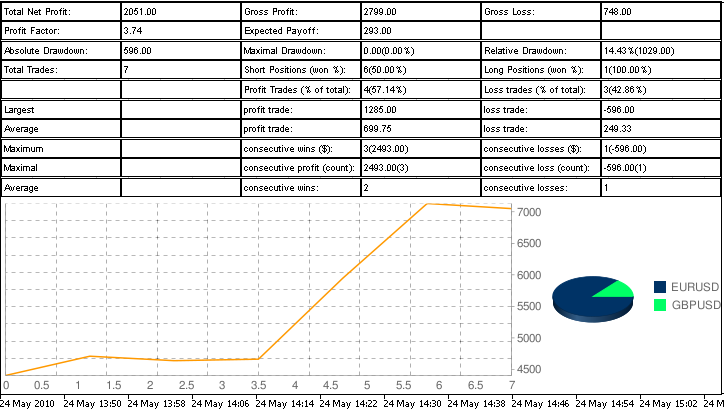Figure 3. The final view of the table

### Conclusion

Dear reader, I hope that in reading this article, you will find something new for yourselves. I have tried to open before you all of the potentialities of such a wonderful instrument as the Standard Library, because it is provides convenience, speed, and high quality of performance. Of course, you need to have some knowledge of OOP.

Good luck.

To get started, unzip the archive MQL5.rar into the terminal folder, and permit the use of DLL. The archive DLL_Sources.zip contains the source codes of the libraries String_Metrics.dll PNG_to_BMP.dll, they were written by me in the Borland C++ Builder environment with an installed GDI.

Translated from Russian by MetaQuotes Software Corp.
Original article: https://www.mql5.com/ru/articles/102

Attached files |
infoboard.zip (200 KB)
dll_sources.zip (1.62 KB)
infoboard-doc-en.zip (1836.47 KB)
Last comments | Go to discussion (3)| 1 Dec 2010 at 13:51

Евгений

Very interesting and potentially very very useful.

Thank you for you effort.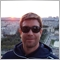| 14 Feb 2012 at 14:05

Hi,

thanks for the good example. the problem I have is that after the last MT5 update the winnet functions are not working. is showing an access violation error. please let me know if you have overcome this error.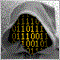| 11 Mar 2013 at 15:34

<vcl.h> is specifity of Borland ? i dont compile because i don t have this file!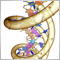Genetic Algorithms - It's Easy!

In this article the author talks about evolutionary calculations with the use of a personally developed genetic algorithm. He demonstrates the functioning of the algorithm, using examples, and provides practical recommendations for its usage.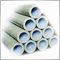A DLL-free solution to communicate between MetaTrader 5 terminals using Named Pipes

The article describes how to implement Interprocess Communication between MetaTrader 5 client terminals using named pipes. For the use of the named pipes, the CNamedPipes class is developed. For the test of its use and to measure the connection throughput, the tick indicator, the server and client scripts are presented. The use of named pipes is sufficient for real-time quotes.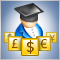Creating an Expert Advisor, which Trades on a Number of Instruments

The concept of diversification of assets on financial markets is quiet old, and has always attracted beginner traders. In this article, the author proposes a maximally simple approach to a construction of a multi-currency Expert Advisor, for an initial introduction to this direction of trading strategies.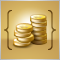Functions for Money Management in an Expert Advisor

The development of trading strategies primarily focuses on searching for patterns for entering and exiting the market, as well as maintaining positions. If we are able to formalize some patterns into rules for automated trading, then the trader faces the question of calculating the volume of positions, the size of the margins, as well as maintaining a safe level of mortgage funds for assuring open positions in an automated mode. In this article we will use the MQL5 language to construct simple examples of conducting these calculations.